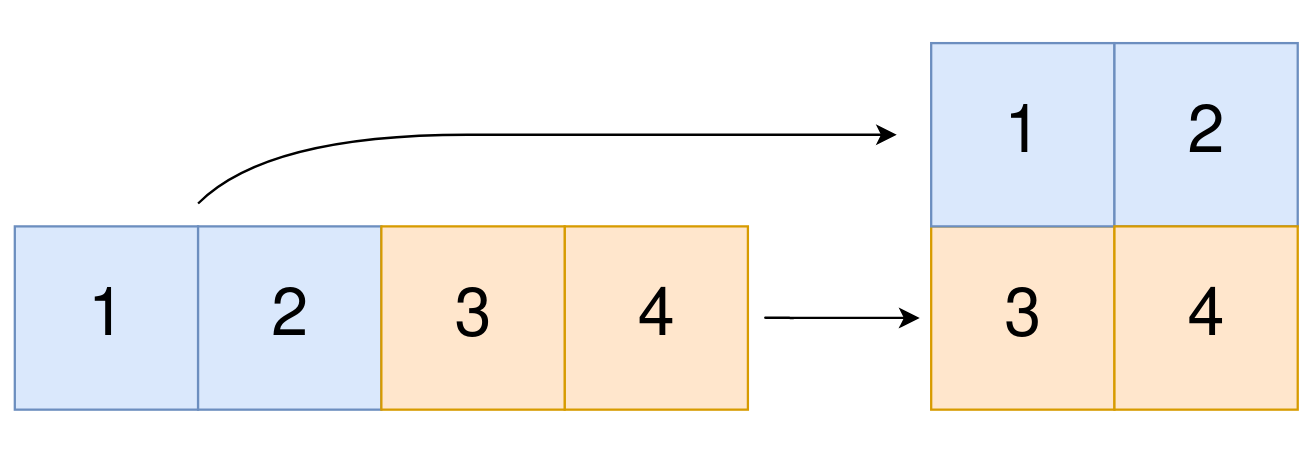Pankaj Tanwar
Published on

# Convert 1D Array Into 2D Array.

Authors
•Name
Pankaj Tanwar
@the2ndfloorguy

Howdy-ho!

I welcome you all to yet another amazing day (btw its' day 28) of my coding diary. It has been a phenomenal journey in terms of solving a challenge and keeping myself disciplined to some extent.

Well, let's boogie!

Problem of the day - Convert 1D Array Into 2D Array

Tag - Easy

You are given a 0-indexed 1-dimensional (1D) integer array original, and two integers, m and n. You are tasked with creating a 2-dimensional (2D) array with m rows and n columns using all the elements from original.

The elements from indices 0 to n - 1 (inclusive) of original should form the first row of the constructed 2D array, the elements from indices n to 2 * n - 1 (inclusive) should form the second row of the constructed 2D array, and so on.

Return an m x n 2D array constructed according to the above procedure, or an empty 2D array if it is impossible.

Example 1:Input: original = [1,2,3,4], m = 2, n = 2 Output: [[1,2],[3,4]]

The problem statement is pretty much clear at first glance itself. I need to convert a given 1D array to 2D of given dimensions.

This statement immediately brought up a very small and quick technique to solve this problem. How about calculating the expected index of each element in the result 2D array? It would be pretty neat to implement and it should work.

Without wasting any further seconds, I coded the solution.

class Solution {public:    vector<vector<int>> construct2DArray(vector<int>& original, int m, int n) {        if(m * n != original.size()) {            return {};        }        vector<vector<int>> res(m, vector<int>(n,0));                for(int i=0;i<original.size();i++) {            res[i/n][i%n] = original[i];        }        return res;    }};

A couple of things I learned from this

• Use {} to return an empty vector in C++
• Initializing 2D array of given dimensions, with 0 as default value can be done by vector<vector<int>> res(m, vector<int>(n,0));

Time Complexity - O(n) (n is the length of original array) Space Complexity - O(1) (No extra space is being used)

I welcome your suggestions to improve it further!

Thanks for being part of my daily-code-workout journey. As always, if you have any thoughts about anything shared above, don't hesitate to reach out.

You might like previous editions of my coding diary